Electron. J. Differential Equations, Vol. 2020 (2020), No. 56, pp. 1-17.

### Positive solutions for asymptotically 3-linear quasilinear Schrodinger equations Guofa Li, Bitao Cheng, Yisheng Huang

Abstract: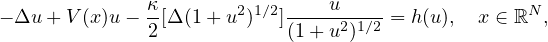where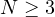,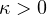is a parameter,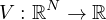is a given potential. The nonlinearity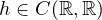is asymptotically 3-linear at infinity. We obtain the nonexistence of a least energy solution and the existence of a positive solution, via the Pohozaev manifold and a linking theorem. Our results improve recent results in [4, 22].Guofa Li College of Mathematics and Statistics Qujing Normal University Qujing 655011, China email liguofa2013@163.com Bitao Cheng College of Mathematics and Statistics Qujing Normal University Qujing 655011, China email: chengbitao2006@126.com Yisheng Huang Department of Mathematics Soochow University Suzhou 215006, China email: yishengh@suda.edu.cn